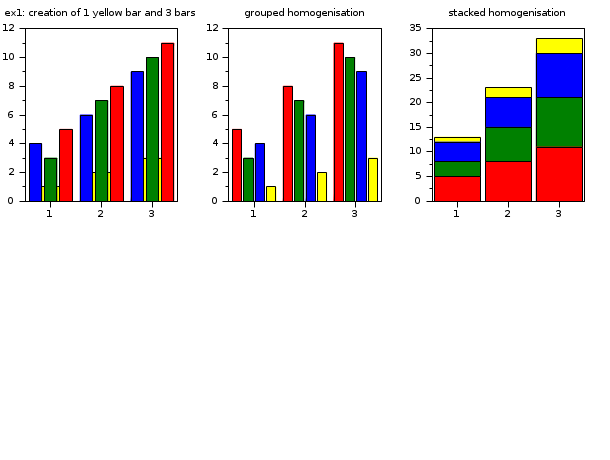Scilab Home page | Wiki | Bug tracker | Forge | Mailing list archives | ATOMS | File exchange
Scilab 5.5.0
Change language to: Français - Português - 日本語 - Русский

See the recommended documentation of this function

Scilab Help >> Graphics > bar_histogram > barhomogenize

# barhomogenize

homogenize all the bars included in the current working axes

### Calling Sequence

```barhomogenize()
barhomogenize([h[,'style'[,'width']]])```

### Arguments

h

an axes handle, (default: h=gca() ).

style

a string, 'grouped' or 'stacked' (default: 'grouped').

width

(optional), a real scalar, defines the width (a percentage of the available room) for the bar (default: 0.8).

### Description

If there are several bar calls, the `barhomogenize` function allows to homogenize the width and style of all bars (i.e which has the `polyline_style` type 6) included in the current working axes. These bars must have the same x data.

`barhomogenize( ) :` takes the default values, h=gca(), width=0.8, style='grouped'.

`barhomogenize(h,...) :` defines the current axes where the drawing is performed.

`barhomogenize(...,width,...) :` defines the width of the bar(s) in percentage (generally: 0<width<=1).

`barhomogenize(...,style,...) :` defines how the bars are drawn. The 'grouped' option allows to center the M polylines versus each components of x, and the 'stacked' option allows to stack them.

### Examples

```// First example: creation of 1 yellow bar (i.e 1 polyline with polyline_style=6) and 3 bars (i.e 3 polylines with polyline_style=6)
subplot(2,3,1)
xtitle('ex1: creation of 1 yellow bar and 3 bars')
x=1:3;
y1=1:3;
y2=[4 3 5;6 7 8;9 10 11];
bar(x,y1,'yellow');bar(x,y2);

// grouped homogenization of these 4 bars
subplot(2,3,2)
xtitle('grouped homogenization')
x=1:3;
y1=1:3;
y2=[4 3 5;6 7 8;9 10 11];
bar(x,y1,'yellow');bar(x,y2);
barhomogenize();

// stacked homogenization of the 4 bars
subplot(2,3,3)
xtitle('stacked homogenization')
x=1:3; y1=1:3; y2=[4 3 5;6 7 8;9 10 11];
bar(x,y1,'yellow');bar(x,y2);
barhomogenize('stacked',1);``````// Second example : creation of 1 red bar (i.e 1 polyline with polyline_style=6) and 2 polylines with type=1 (calling plot function)
subplot(2,3,4)
xtitle('ex2: creation of 1 bar and 2 polylines')
x=1:10;
y=sin(x)/2;
bar(x,y,'red')
x1=1:10;
y1=[sin(x);cos(x)]
plot(x1',y1')

// modify the polyline_style type of the second polyline from plot (this polyline becomes a bar)
subplot(2,3,5)
xtitle('transformation of the second polyline to bar')
x=1:10;
y=sin(x)/2;
bar(x,y,'red')
x1=1:10;
y1=[sin(x);cos(x)]
plot(x1',y1')
e=gce();
e2=e.children(2);
e2.polyline_style=6;

// homogenization of the first bar (from bar function) and second bar (from the modification).
subplot(2,3,6)
xtitle('grouped homogenization')
x=1:10;
y=sin(x)/2;
bar(x,y,'red')
x1=1:10;
y1=[sin(x);cos(x)]
plot(x1',y1')
e=gce();
e2=e.children(2);
e2.polyline_style=6;
barhomogenize();
// change the style and the width
//barhomogenize('stacked',0.5);
//barhomogenize('stacked',1);```